# FORM THREE MATHEMATICS STUDY NOTES TOPIC 1-2.

0
731TOPIC 1: RELATIONS

Normally
relation deals with matching of elements from the first set called
DOMAIN with the element of the second set called RANGE.
A relation “R” is the rule that connects or links the elements of one set with the elements of the other set.
Some examples of relations are listed below:
1. “Is a brother of “
2. “Is a sister of “
3. “Is a husband of “
4. “Is equal to “
5. “Is greater than “
6. “Is less than “
Normally
relations between two sets are indicated by an arrow coming from one
element of the first set going to the element of the other set.
Relations Between Two Sets
Find relations between two sets
The relation can be denoted as:
R = {(a, b): a is an element of the first set, b is an element of the second set}
Consider the following table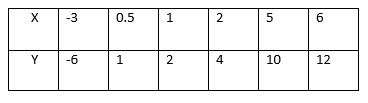This
is the relation which can be written as a set of ordered pairs {(-3,
-6), (0.5, 1), (1, 2), (2, 4), (5, 10), (6, 12)}. The table shows that
the relation satisfies the equation y=2x. The relation R defining the
set of all ordered pairs (x, y) such that y = 2x can be written
symbolically as:
R = {(x, y): y = 2X}.
Relations Between Members in a Set
Find relations between members in a set
Which of the following ordered pairs belong to the relation {(x, y): y>x}?
(1, 2), (2, 1), (-3, 4), (-3, -5), (2, 2), (-8, 0), (-8, -3).
Solution.
(1, 2), (-3, 4), (-8, 0), (8,-3).
Relations Pictorially
Demonstrate relations pictorially
For
example the relation ” is greater than ” involving numbers 1,2,3,4,5
and 6 where 1,3 and 5 belong to set A and 2,4 and 6 belong to set B can
be indicate as follows:-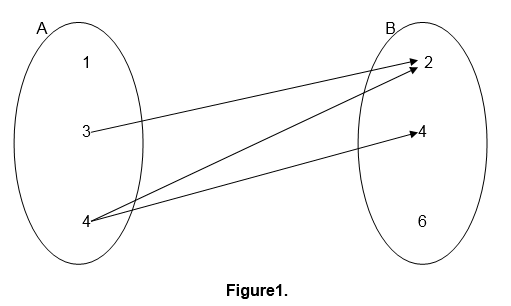This kind of relation representation is referred to as pictorial representation.
Relations
can also be defined in terms of ordered pairs (a,b) for which a is
related to b and a is an element of set A while b is an element of set
B.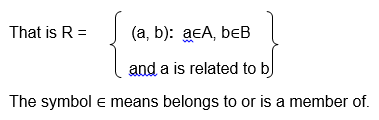For
example the relation ” is a factor of ” for numbers 2,3,5,6,7 and 10
where 2,3,5 and 6 belong to set A and 6,7 and 10 belong to set B can be
illustrated as follows:-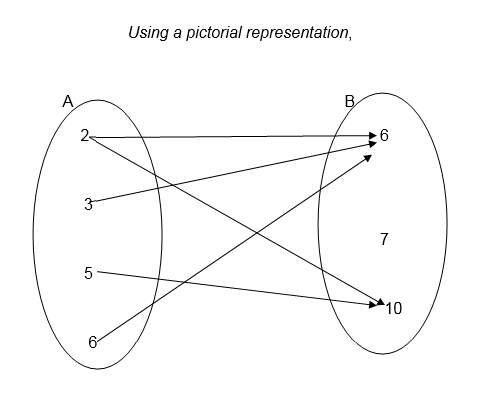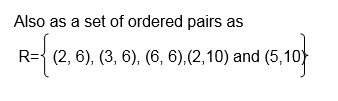Example 1
<!–
[if !supportLists]–>1. Draw an arrow diagram to illustrate the
relation which connects each element of set A with its square.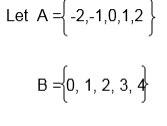Solution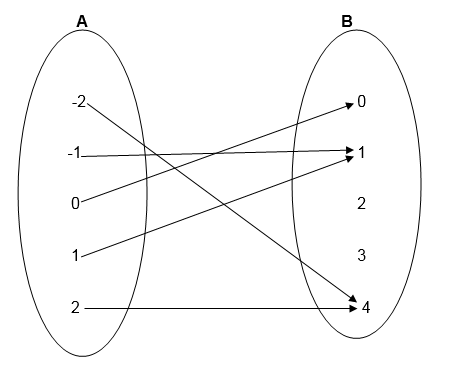Example 2
Using
the information given in example 1, write down the relation in set
notation of ordered pairs. List the elements of ordered pairs.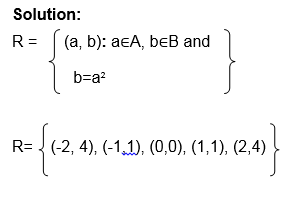Example 3
As we,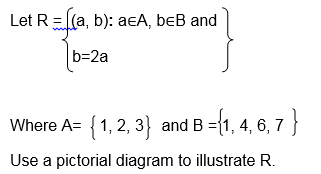Solution;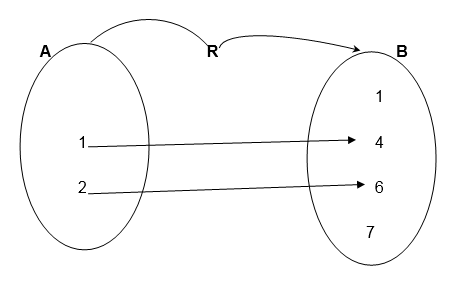Example 4
Let X= {2, 3, 4 } and Y= {3 ,4, 5}
Draw an arrow diagram to illustrate the relation ” is less than”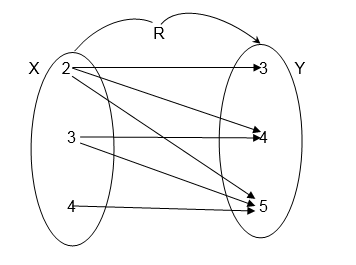Exercise 1
Let P= {Tanzania, China, Burundi, Nigeria}
Draw a pictorial diagram between P and itself to show the relation
“Has a larger population than”
2. Let A = 9,10,14,12 and B = 2,5,7,9 Draw an arrow diagram between A and B to illustrate the relation ” is a multiple of”
3.Let A = mass, Length, time and
B = {Centimeters, Seconds, Hours, Kilograms, Tones}
Use the set notation of ordered pairs to illustrate the relation “Can be measured in”
4.
A group people contain the following; Paul Koko, Alice Juma, Paul
Hassan and Musa Koko. Let F be the set of all first names, and S the set
of all second names.
Draw an arrow diagram to show the connection between F and S
5. Let R={ (x, y): y=x+2}
Where x∈A and A ={ -1,0,1,2}
and y∈B, List all members of set B
Exercise 2
1. Let the relation be defined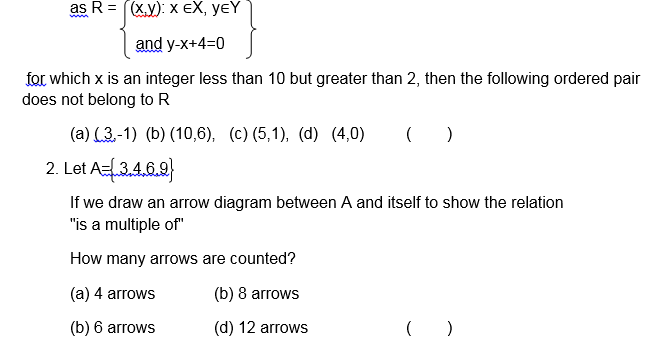Consider the following pictorial diagram representing a relation R.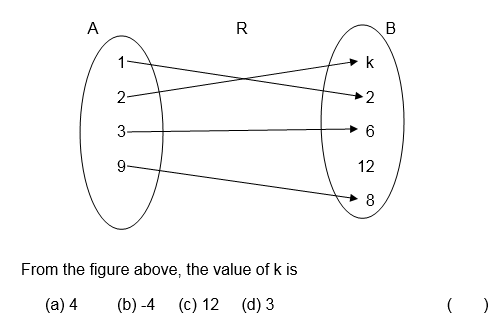Let the relation R be defined asA relation R on sets a and B where A = 1,2,3,4,5 and B = 7,8,9,10,11,12 is defined as ” is a factor of “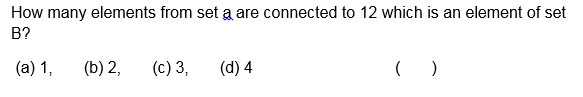TOPIC 2: FUNCTIONS

Normally
relation deals with matching of elements from the first set called
DOMAIN with the element of the second set called RANGE.
Definitions:
A
function is a relation with a property that for each element in the
domain there is only one corresponding element in the range or co-
domain
Therefore functions are relations but not all relations are functions
The Concept of a Functions Pictorially
Explain the concept of a functions pictorially
Example 1
Which of the following relation are functions?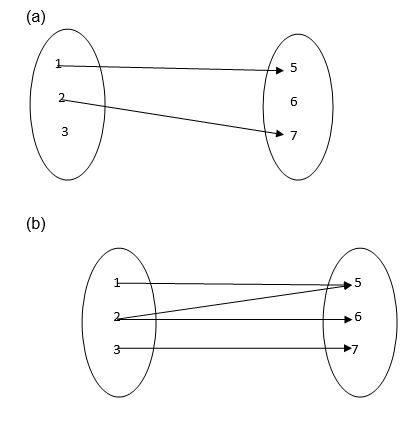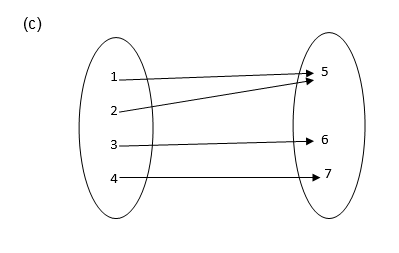Solution
1. It is not a function since 3 and 6 remain unmapped.
2. It is not a function because 2 has two images ( 5 and 6)
3. It is a function because each of 1, 2, 3 and 4 is connected to exactly one of 5, 6 or 7.
Functions
Identify functions
TESTING FOR FUNCTIONS;
If
a line parallel to the y-axis is drawn and it passes through two or
more points on the graph of the relation then the relation is not a
function.
If it passes through only one point then the relation is a function
Example 2
Identify each of the following graphs as functions or not.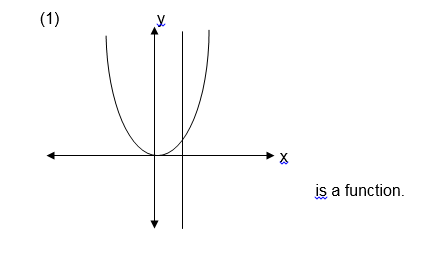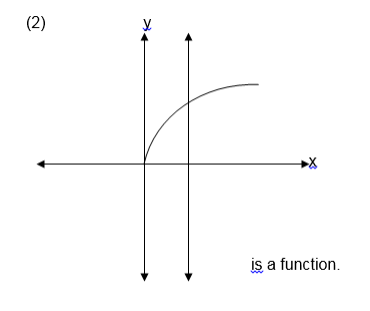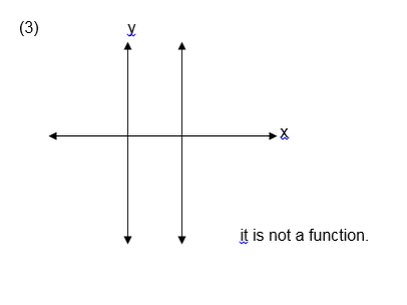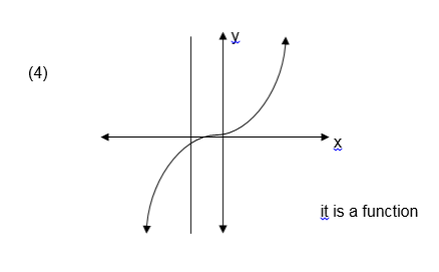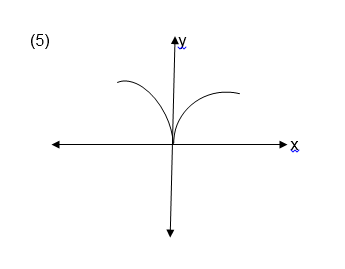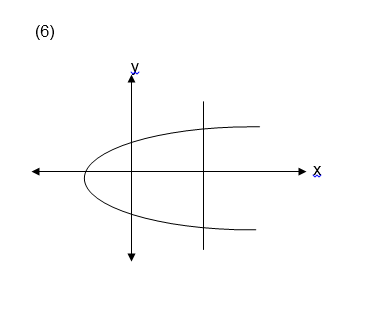Exercise 1
1. Which of the following relations are functions?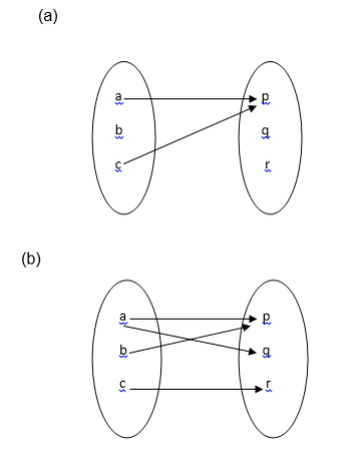2. Let A = {1, 2, 3, 4, 5, 6, 7, 8, 9, 10}
and B ={ 2, 3, 5, 7 }
Draw an arrow diagram to illustrate the relation “ is a multiple of ‘ is it a function ? why?
3. let A = {1,-1 ,2,-2} and
B = {1, 2, 3, 4 } which of the following relations are functions ?
1. { ( x , y ) : x < y }
2. { ( x , y ) : x > y}
3. { ( x , y ) : y = x2}